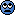# Derivations for Schrodinger's equations for potential step

• space-time
In summary, the conversation discussed the different versions of the Schrodinger equation for a step potential and how they were derived. It was concluded that these versions are just algebraically manipulated versions of the original equation and are not necessary for solving it. However, these manipulations do make solving the equation simpler and more manageable.

#### space-time

I have been studying potential steps and barriers as well as reflection and transmission coefficients and how to derive them. Most of it makes sense to me except for one thing:

As we know, the normal Schrodinger equation is:

(-ħ2/2m) (∂2Ψ/∂x2) + v(x)Ψ = EΨ

For a step potential however, my book and web resource both say that for the boundary conditions:

v(x)= 0 for x < 0 and V0 for x ≥ 0

the Schrodinger equations are:

(∂2Ψ1/∂x2) + K12Ψ1(x) = 0

and
(∂2Ψ2/∂x2) + K22Ψ2(x) = 0

where K1 = squrt(2mE) / ħ and K2 = squrt(2m(E- V0)) / ħ

(the plus sign in the second one changes into a minus when the particle doesn't have enough energy to overcome the step).

Where/How exactly did Schrodinger get these step potential equations from the original one? The step potential equations don't even seem to have the (-ħ2/2m) term in front. Can someone please explain to me why step potentials seem to have different Schrodinger equations than the normal one?

This is just basic algebra, giving ##K_1## as an example, and you should work out ##K_2## for yourself:

$$\frac{-\hbar^2}{2m}\frac{\partial^2}{\partial x^2}\psi=E\psi$$ $$\frac{\partial^2}{\partial x^2}\psi=-\frac{2m}{\hbar^2}E\psi$$ $$\frac{\partial^2}{\partial x^2}\psi+\frac{2mE}{\hbar^2}\psi=0$$ $$\frac{\partial^2}{\partial x^2}\psi+K_1^2\psi=0$$

Matterwave said:
This is just basic algebra, giving ##K_1## as an example, and you should work out ##K_2## for yourself:

$$\frac{-\hbar^2}{2m}\frac{\partial^2}{\partial x^2}\psi=E\psi$$ $$\frac{\partial^2}{\partial x^2}\psi=-\frac{2m}{\hbar^2}E\psi$$ $$\frac{\partial^2}{\partial x^2}\psi+\frac{2mE}{\hbar^2}\psi=0$$ $$\frac{\partial^2}{\partial x^2}\psi+K_1^2\psi=0$$

I see. Thank you. It is just simple algebra. This does bring up the question however of why that was necessary in the first place. Why didn't he just leave the equation as it originally was (since these other versions are just algebraically manipulated versions of the original)? After all, the original version is just as simple to solve as the other versions. I have a hypothesis as to why this manipulation was necessary (it has to do with being able to normalize the solutions). I am not 100% sure however that this is the reason.

space-time said:
I see. Thank you. It is just simple algebra. This does bring up the question however of why that was necessary in the first place. Why didn't he just leave the equation as it originally was (since these other versions are just algebraically manipulated versions of the original)? After all, the original version is just as simple to solve as the other versions. I have a hypothesis as to why this manipulation was necessary (it has to do with being able to normalize the solutions). I am not 100% sure however that this is the reason.

No, you can solve it without doing any algebraic manipulations. And this has nothing to do with normalization. But if you do these manipulations, it just makes things a little simpler when you go ahead and solve it.

When you finally start writing out the solutions and manipulating them algebraically, would you rather wrestle with things like ##e^{ik_1 x}## and ##e^{ik_2 x}## or with things like ##e^{i\sqrt{2mE}x/\hbar}## and ##e^{i\sqrt{2m(E-V_0)}x/\hbar}## ?